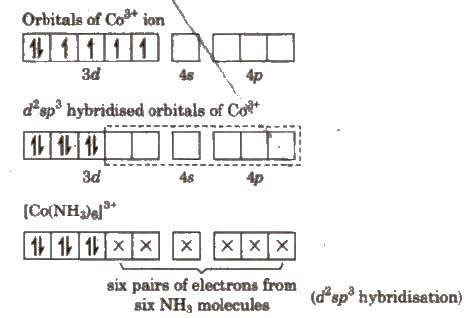# Question 709e7

Apr 9, 2017

An inner orbital complex uses $\text{d}$ orbitals from the $\left(n \text{-1}\right)$ shell to form ${\text{d"^2"sp}}^{3}$ hybrid orbitals for bonding to the ligands.

#### Explanation:

An outer orbital complex uses $\text{d}$ orbitals from the $\left(n\right)$ shell to form ${\text{sp"^3"d}}^{2}$ hybrid orbitals for bonding.

Inner orbital complexes

["Co"("NH"_3)_6]^"3+" is an inner orbital complex.

The electron configuration of $\text{Co"^"3+}$ is ${\text{[Ar] 3d}}^{6}$.The $\text{3d, 4s}$ and $\text{4p}$ orbitals are close in energy, so the ion can easily form six ${\text{d"^2"sp}}^{3}$ hybrid orbitals for bonding to the ${\text{NH}}_{3}$ ligands.

This is an inner orbital complex because it uses the "inner" $\text{3d}$ orbitals for bonding.

Outer orbital complexes

["CoF"_6]^"3-"# is an outer orbital complex.Here, the empty $\text{4s, 4p}$ and two of the $\text{4d}$ orbitals can form six ${\text{sp"^2"d}}^{3}$ hybrid orbitals for bonding to the $\text{F"^"-}$ ligands.

This is an outer orbital complex because it uses the "outer" $\text{4d}$ orbitals for bonding.

For an excellent explanation of inner and outer orbital complexes, see this Socratic answer.